## Sizing a Pump(Metric Units)

Summary

This example will walk you through a simple calculation to size a pump.

A pump is to be used to transfer water from a supply reservoir to a holding reservoir at the top of a hill. The system consists of a supply reservoir, a pump, a discharge reservoir, and two pipes.

The first pipe from the supply reservoir to the pump is 3 meters long, and the second pipe leading from the pump to the discharge reservoir is 300 meters long. Both pipes are 4 inch, Steel - ANSI, STD (Schedule 40) pipes. Constant fluid properties are assumed.

The supply reservoir has a liquid surface pressure of 0 barG (0 kPa(g)), and the liquid surface is at an elevation of 3 meters. The discharge reservoir has a liquid surface pressure of 0 barG (0 kPa(g)), and the liquid surface is at an elevation of60 meters. The pipes for both reservoirs connect to the reservoirs at a depth of 3 meters.

What is the head requirement for a pump to supply flow in this system at 115 m3/hr?

Topics Covered

• Specifying a pump in the Pump Properties Window

• Defining pipe fittings and losses lumped into a pipe

• Sizing a pump

• Entering pump curve data

Required Knowledge

This example assumes the user has already worked through the Beginner: Three Reservoir Problem example, or has a level of knowledge consistent with that topic. You can also watch the AFT Fathom Quick Start Video Tutorial Series on the AFT website, as it covers the majority of the topics discussed in the Three-Reservoir Problem example.

Model File

This example uses the following file, which is installed in the Examples folder as part of the AFT Fathom installation:

Step 1. Start AFT Fathom

From the Start Menu choose the AFT Fathom 12 folder and select AFT Fathom 12.

To ensure that your results are the same as those presented in this documentation, this example should be run using all default AFT Fathom settings, unless you are specifically instructed to do otherwise.

Step 2. Define the Fluid Properties Group

1. Open Analysis Setup from the toolbar or from the Analysis menu.

2. Open the Fluid panel then define the fluid:

1. Fluid Library = AFT Standard

2. Fluid = Water (liquid)

1. After selecting, click Add to Model

3. Temperature = 21 deg. C

Step 3. Define the Pipes and Junctions Group

At this point, the first two groups are completed in Analysis Setup. The next undefined group is the Pipes and Junctions group. To define this group, the model needs to be assembled with all pipes and junctions fully defined. Click OK to save and exit Analysis Setup then assemble the model on the workspace as shown in the figure below.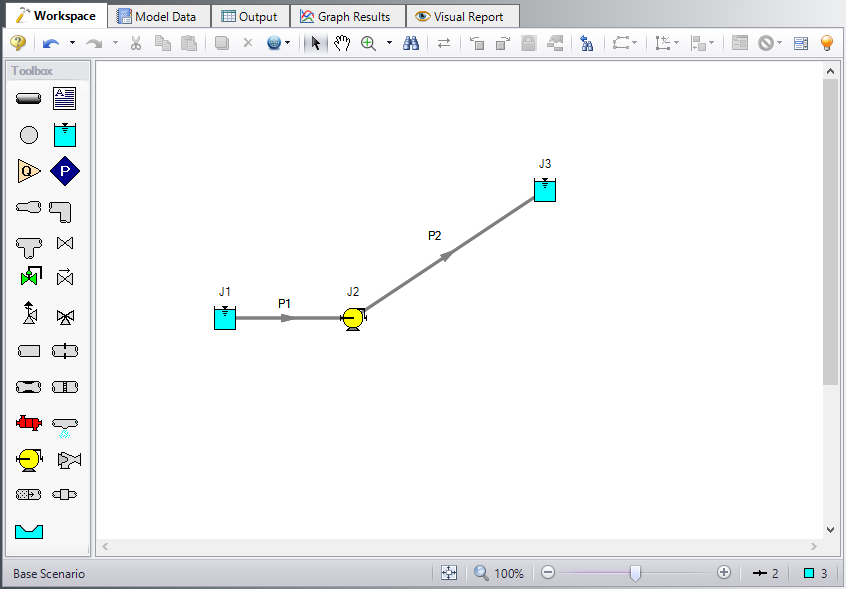Figure 1: Workspace for Sizing a Pump Example

The system is in place but now we need to enter the properties of the objects. Double-click each pipe and junction and enter the following properties. The required information is highlighted in blue.

Pipe Properties

1. Pipe Model tab

1. Pipe Material = Steel - ANSI

2. Pipe Geometry = Cylindrical Pipe

3. Size = 4 inch

4. Type = STD (schedule 40)

5. Friction Model Data Set = Standard

6. Lengths =

Pipe Length (meters)
1 3
2 300

The pipe model also allows for fittings and losses like valves or elbows. Select the Fittings & Losses tab for P2, and type in 25 for the Total K Factor. Now click OK to close the Pipe Properties window and accept your changes.

Note: A red ampersand (&) will now appear next to the pipe number for pipe P2. This indicates that Fittings & Losses are entered in the Pipe Properties window. This symbol can be edited or hidden in User Options.

Junction Properties

1. J1 Reservoir

1. Name = Lower Reservoir

2. Liquid Surface Elevation = 3 meters

3. Liquid Surface Pressure = 0 barG (0 kPa (g))

4. Pipe Depth = 3 meters

2. J2 Pump

1. Inlet Elevation = 0 meters

2. Pump Model = Centrifugal (Rotodynamic)

3. Analysis Type = Sizing

4. Parameter = Volumetric Flow Rate

5. Fixed Flow Rate = 115 m3/hr

3. J3 Reservoir

1. Name = Upper Reservoir

2. Liquid Surface Elevation = 60 meters

3. Liquid Surface Pressure = 0 barG (0 kPa (g))

4. Pipe Depth = 3 meters

ØTurn on Show Object Status from the View menu to verify if all data is entered. If so, the Pipes and Junctions group in Analysis Setup will have a check mark. If not, the uncompleted pipes or junctions will have their number shown in red. If this happens, go back to the uncompleted pipes or junctions and enter the missing data.

Step 4. Run the Model

Click Run Model on the toolbar or from the Analysis menu. This will open the Solution Progress window. This window allows you to watch as the AFT Fathom solver converges on the answer. This model runs very quickly. Now view the results by clicking the Output button at the bottom of the Solution Progress window.

Step 5. Examine the Output

The Output window (Figure 2) contains all the data that was specified in the output control window. Because we were interested in the pump requirements for this system, select the Pump Summary tab in the General (top) window. The pump data can also be found in the Junction (bottom) window. We are primarily interested in the pump head (or pressure) requirement. This information can be found in the Pump Summary window.

For this system, the head requirement of the pump is 116.3 meters. Because the difference in elevation of the reservoirs was 57 meters this is the minimum head rise regardless of flow rate or pipe size. Coincidentally, the friction loss was also approximately 59 meters. This can be seen in the dH column of the Pipe output window.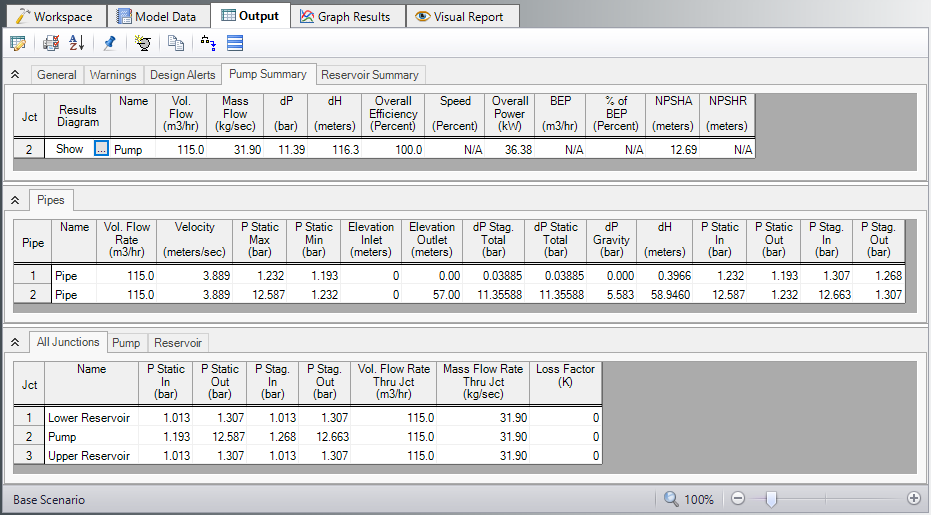Figure 2: Output window for Pump Sizing Example

Step 6. Add a Pump Curve

Now that the pump head requirement has been identified as 116.3 meters at the design flow rate of 115 m3/hr, a pump of the correct size can be purchased to meet this requirement. Once the actual pump has been identified, the pump characteristics can be added to the pump by adding the pump curve data to the pump junction.

Open the Pump Properties window and enter the following data:

1. Pump Model = Centrifugal (Rotodynamic)

2. Analysis Type = Pump Curve

3. Enter Curve Data

1. Flow Q Parameter = Volumetric

m3/hr meters
0 122
115 116
230 76
1. Curve Fit Order = 2

2. Click Generate Curve Fit Now

3. Click OK

Note: This is a situation where a user could create a new scenario using the Scenario Manager to examine a what-if situation, without disturbing the basic model. For an example that illustrates how to use the Scenario Manager, see the Water to Housing Project example.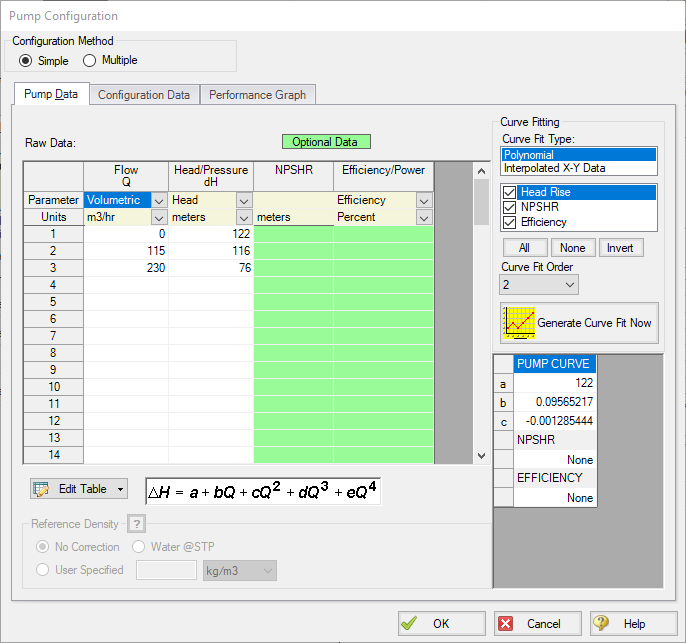Figure 3: The Pump Configuration Window is used to enter pump curve data

Step 7. Re-evaluate the model

Re-run this model. Examine the Pump Summary in the General Output Section. The output (Figure 4) shows the pump operating at a flow rate of 114.7 m3/hr, and a head rise of 116.1 meters, which is acceptably close to the previous sizing calculation.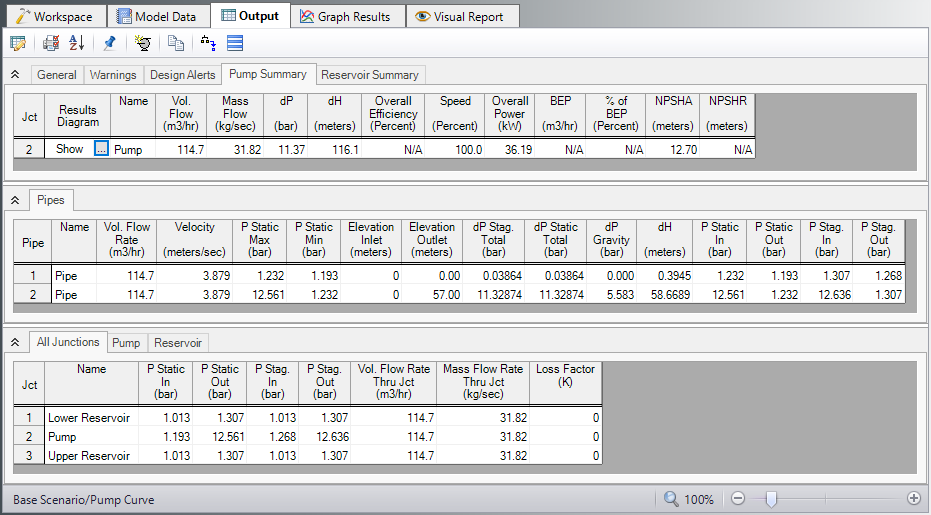Figure 4: Output when running with a pump curve

Change to the Graph Results window (e.g., using the Windows menu), and click on the Pump vs. System tab (Figure 5) in the Graph Parameters menu on the Quick Access Panel. Click Generate to graph a pump vs. system curve, as shown in Figure 6.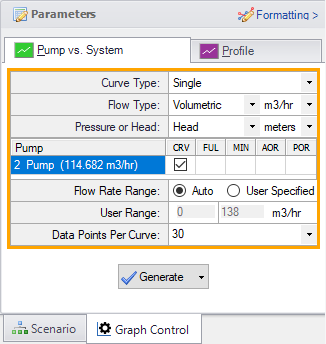Figure 5: Select Graph Data for creating a pump vs. system curve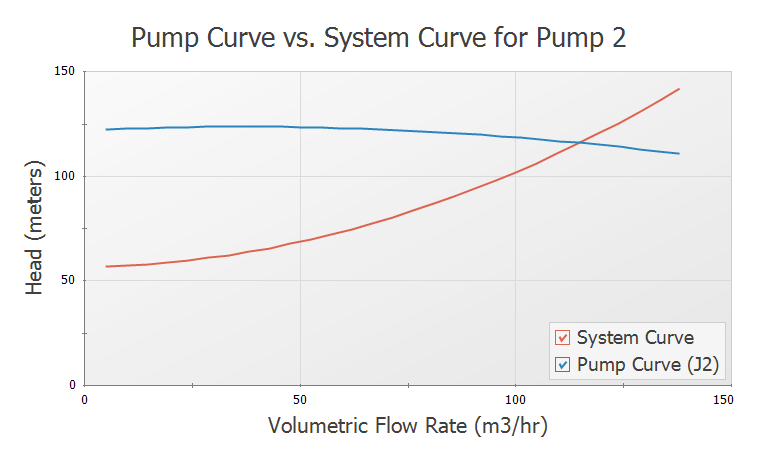Figure 6: Pump vs. system curve using selected pump

Conclusion

AFT Fathom can be used to size pumps. Once the actual pump has been selected, the model can be modified to include the actual pump curve data. Then the actual system properties and behavior can be calculated.